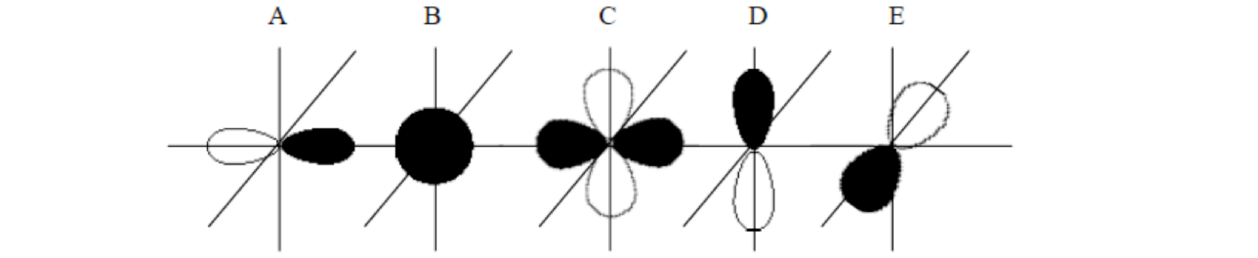# Problem: For the first three questions, consider the bottom set of five orbitalsHow many nodes are displayed in orbital ‘D’?a. 0b. 1c. 2d. 3e. 4What is the best label for orbital ‘A’?a. 1sb. 2sc. 2pd. 3se. 3pWhich orbital has the highest energy?a. Ab. Bc. Cd. De. E

###### FREE Expert Solution
100% (337 ratings)
###### Problem Details

For the first three questions, consider the bottom set of five orbitals

How many nodes are displayed in orbital ‘D’?

a. 0

b. 1

c. 2

d. 3

e. 4

What is the best label for orbital ‘A’?

a. 1s

b. 2s

c. 2p

d. 3s

e. 3p

Which orbital has the highest energy?

a. A

b. B

c. C

d. D

e. E